### Temperature – Concepts, Scales, Fixed Points and Measurements

Temperature
Is an intensive property independent of size and mass of the system. It may be defined as the –

• degree of hotness or coldness of a body or an environment

• driving force or potential causing the flow of energy as heat

• measure of the mean kinetic energy of the molecules of a system

• parameter which determines whether or not a system is in thermal equilibrium

Thermometry
It may be defined as the act of measuring temperature with accuracy and precision. The Zeroth law of thermodynamics is the basis of Temperature Measurement. In order to obtain a quantitative measure of temperature a reference body is used and a certain physical characteristic of this body which changes with temperature is selected. The changes in the selected characteristics are called as an indication of change in temperature. The selected characteristics are called “Thermometric Property” and the reference body which is used in the determination of temperature is called “Thermometer”Picture: Mercury Glass Thermometer

For example: Mercury Glass thermometer – A very common thermometer which consists of a small amount of mercury in an evacuated capillary tube. In this extension of the mercury in the tube is used as the thermometric property.

There are five different kinds of thermometer with its own thermometric property as below –

 Sl NO. Thermometer Thermometric property 1 Constant volume gas thermometer Pressure 2 Constant pressure gas thermometer Volume 3 Electrical resistance thermometer Resistance 4 Thermocouple Thermal emf 5 Mercury in glass thermometer Length

Calibration is then achieved through comparison with established standards. Any one of the following relationship between temperature (t) and the thermometric property () can be utilized to establish a temperature scale –The constants appearing in these relations can be determined from any two fixed thermometric points, e.g., ice point and steam point

Temperature Scales
A quantitative measurement of the temperature of a system requires reference to some datum plane or reference condition, and the establishment of a suitable temperature unit. Many temperature scales and reference points have been proposed; the important ones are –

1. Constant Pressure Gas Thermometer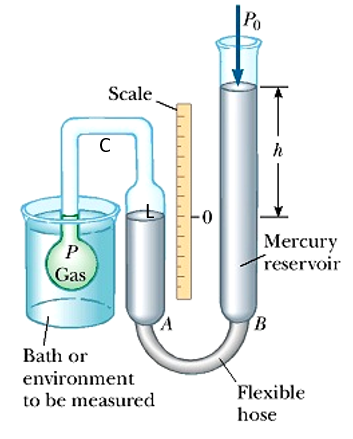Picture: Gas Thermometer

A schematic diagram of a constant pressure gas thermometer is given in figure. A small amount of gas is enclosed in bulb ‘P’ which is in communication via the capillary tube ‘C’ with one limb of the mercury manometer ‘A’. The other limb of the mercury manometer ‘B’ is open to the atmosphere and can be moved vertically to adjust the mercury level so that the mercury just touches tip ‘L’ of the capillary to keep ‘h’ constant. The volume in the bulb is used as a thermometric property.

In a constant pressure gas thermometer, the mercury levels have to be adjusted to keep ‘h’ constant and the volume of gas V which would vary with the temperature of the system becomes the thermometric property.

When the bulb is brought in contact with the system whose temperature is to be measured the bulb in course of time comes into thermal equilibrium with the system. The gas in the bulb expands on being heated pushing the mercury downward. The flexible limb of the manometer is then adjusted so that the mercury again touches the lip  ‘L’ and the difference in mercury level ‘h’ remains constant as of initial value and the volume of the gas in the bulb is estimated. Since the volume of trapped gas varies, then from the ideal gas equation-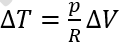I.e. the temperature increase is proportional to the observed volume increase

2.  International Practical Temperature Scale

An International temperature scale was adopted at the “7th General Conference on Weights and Measures held in 1927”. It was not to replace the Celsius or ideal gas scales but to provide an experimental basis scale that can be easily and rapidly used to calibrate scientific and industrial instruments to indicate temperatures as close as possible to the Kelvin thermodynamic scale. The international practical scale agrees with the Celsius scale at the defining fixed points listed below-

 Sl No. Fixed Points Tempearture °C 1 Temperature of equilibrium between liquid and vapour oxygen Oxygen point -182.97 2 Temperature of equilibrium between ice and air saturated water Ice point 0.000 3 Temperature of equilibrium between liquid water and its vapour Steam point 100.000 4 Temperature of equilibrium between liquid sulfur and its vapour Sulfur point 444.600 5 Temperature of equilibrium between solid and liquid silver Silver point 960.800 6 Temperature of equilibrium between solid and liquid gold Gold point 1063.000

For interpolation between these primary fixed points and numerous other secondary fixed points, a thermometer is specified with an equation to establish relationship between thermometric property and temperature. The method of evaluating constants in the correlation is also specified.

For example-

• From -190°C to 0°C

A platinum resistance thermometer with a platinum wire whose diameter must lie between 0.05mm and 0.20mm is used and the temperature is defined by a polynomial of the form –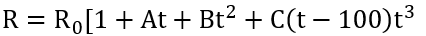Where: the constants Ro, A, B and C are computed by measurements at the ice point, steam point, sulfur point and oxygen point respectively.

• From 0°C to 660°C

A platinum resistance thermometer with a platinum wire whose diameter must lie between 0.05mm and 0.20mm is used and the temperature is defined by –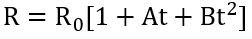Where: the constants Ro, A and B are computed by measurements at the ice point, steam point and sulfur point respectively.

• From 660°C to 1063°C

A thermocouple of one wire which is made of platinum and other one of alloy of 90% platinum and 10% rhodium is used with one junction at 0°C. The diameter of each wire of the thermocouple lies between 0.35 to 0.65mm. The temperature is defined by –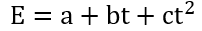Where: the constants a, b and c are computed by measurements at the antimony point, silver point and gold point respectively.

An optical method was adopted for measuring temperatures higher than the gold point. The intensity of radiation of any convenient wavelength is compared with the intensity of radiation of the same wavelength emitted by a black body at the gold point. The temperature is then determined with the help of “Planck’s law of thermal radiation”.